xEscape velocityOverview

In physics
Physics
Physics is a natural science that involves the study of matter and its motion through spacetime, along with related concepts such as energy and force. More broadly, it is the general analysis of nature, conducted in order to understand how the universe behaves.Physics is one of the oldest academic...

, escape velocity is the speed
Speed
In kinematics, the speed of an object is the magnitude of its velocity ; it is thus a scalar quantity. The average speed of an object in an interval of time is the distance traveled by the object divided by the duration of the interval; the instantaneous speed is the limit of the average speed as...

at which the kinetic energy
Kinetic energy
The kinetic energy of an object is the energy which it possesses due to its motion.It is defined as the work needed to accelerate a body of a given mass from rest to its stated velocity. Having gained this energy during its acceleration, the body maintains this kinetic energy unless its speed changes...

plus the gravitational potential energy of an object is zero gravitational potential energy is negative since gravity is an attractive force and the potential is defined to be zero at infinity. It is the speed needed to "break free" from a gravitational field without further propulsion.

For a spherically-symmetric body, escape velocity is calculated by the formula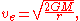where G is the universal gravitational constant
Gravitational constant
The gravitational constant, denoted G, is an empirical physical constant involved in the calculation of the gravitational attraction between objects with mass. It appears in Newton's law of universal gravitation and in Einstein's theory of general relativity. It is also known as the universal...

(G=6.67×10-11 m3 kg-1 s-2), M the mass of the planet, star or other body, and r the distance from the centre of gravity.

In this equation atmospheric friction or air drag, is not taken into account.Unanswered QuestionsDiscussionsWhat are you escaping fromEncyclopedia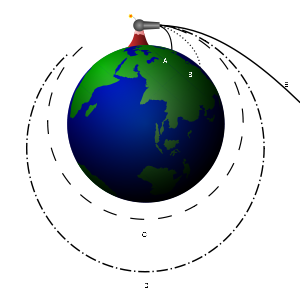In physics
Physics
Physics is a natural science that involves the study of matter and its motion through spacetime, along with related concepts such as energy and force. More broadly, it is the general analysis of nature, conducted in order to understand how the universe behaves.Physics is one of the oldest academic...

, escape velocity is the speed
Speed
In kinematics, the speed of an object is the magnitude of its velocity ; it is thus a scalar quantity. The average speed of an object in an interval of time is the distance traveled by the object divided by the duration of the interval; the instantaneous speed is the limit of the average speed as...

at which the kinetic energy
Kinetic energy
The kinetic energy of an object is the energy which it possesses due to its motion.It is defined as the work needed to accelerate a body of a given mass from rest to its stated velocity. Having gained this energy during its acceleration, the body maintains this kinetic energy unless its speed changes...

plus the gravitational potential energy of an object is zero gravitational potential energy is negative since gravity is an attractive force and the potential is defined to be zero at infinity. It is the speed needed to "break free" from a gravitational field without further propulsion.

For a spherically-symmetric body, escape velocity is calculated by the formulawhere G is the universal gravitational constant
Gravitational constant
The gravitational constant, denoted G, is an empirical physical constant involved in the calculation of the gravitational attraction between objects with mass. It appears in Newton's law of universal gravitation and in Einstein's theory of general relativity. It is also known as the universal...

(G=6.67×10-11 m3 kg-1 s-2), M the mass of the planet, star or other body, and r the distance from the centre of gravity.

In this equation atmospheric friction or air drag, is not taken into account. A rocket moving out of a gravity well does not actually need to attain escape velocity to do so, but could achieve the same result at walking speed with a suitable mode of propulsion and sufficient fuel. Escape velocity only applies to ballistic trajectories.

The term escape velocity
Velocity
In physics, velocity is speed in a given direction. Speed describes only how fast an object is moving, whereas velocity gives both the speed and direction of the object's motion. To have a constant velocity, an object must have a constant speed and motion in a constant direction. Constant ...

is actually a misnomer, as the concept refers to a scalar
Scalar (physics)
In physics, a scalar is a simple physical quantity that is not changed by coordinate system rotations or translations , or by Lorentz transformations or space-time translations . This is in contrast to a vector...

speed which is independent of direction whereas velocity is the measurement of the rate and direction of change in position of an object.

## OverviewA barycentric
Center of mass
In physics, the center of mass or barycenter of a system is the average location of all of its mass. In the case of a rigid body, the position of the center of mass is fixed in relation to the body...

velocity is a velocity of one body relative to the center of mass of a system of bodies. A relative velocity is the velocity of one body with respect to another. Relative escape velocities exist only in systems with two bodies. For systems of two bodies the term "escape velocity" is ambiguous, but it is usually intended to mean the barycentric escape velocity of the less massive body. In gravitational fields "escape velocity" refers to the escape velocity of zero mass test particles relative to the barycenter of the masses generating the field.

The phenomenon of escape velocity is a consequence of conservation of energy
Conservation of energy
The nineteenth century law of conservation of energy is a law of physics. It states that the total amount of energy in an isolated system remains constant over time. The total energy is said to be conserved over time...

. For an object with a given total energy, which is moving subject to conservative force
Conservative force
A conservative force is a force with the property that the work done in moving a particle between two points is independent of the path taken. Equivalently, if a particle travels in a closed loop, the net work done by a conservative force is zero.It is possible to define a numerical value of...

s (such as a static gravity field) it is only possible for the object to reach combinations of places and speeds which have that total energy; and places which have a higher potential energy than this cannot be reached at all.

For a given gravitational potential energy at a given position, the escape velocity is the minimum speed
Speed
In kinematics, the speed of an object is the magnitude of its velocity ; it is thus a scalar quantity. The average speed of an object in an interval of time is the distance traveled by the object divided by the duration of the interval; the instantaneous speed is the limit of the average speed as...

an object without propulsion
Spacecraft propulsion
Spacecraft propulsion is any method used to accelerate spacecraft and artificial satellites. There are many different methods. Each method has drawbacks and advantages, and spacecraft propulsion is an active area of research. However, most spacecraft today are propelled by forcing a gas from the...

needs, to be able to "escape" from the gravity (i.e. so that gravity will never manage to pull it back). For the sake of simplicity, unless stated otherwise, we will assume that the scenario we are dealing with is that an object is attempting to escape from a uniform spherical planet by moving straight away (along a radial line away from the center of the planet), and that the only significant force acting on the moving object is the planet's gravity.

Escape velocity is actually a speed (not a velocity) because it does not specify a direction: no matter what the direction of travel is, the object can escape the gravitational field (though its path may intersect the planet). The simplest way of deriving the formula for escape velocity is to use conservation of energy. Imagine that a spaceship of mass m is at a distance r from the center of mass of the planet, whose mass is M. Its initial speed is equal to its escape velocity,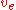. At its final state, it will be an infinite distance away from the planet, and its speed will be negligibly small and assumed to be 0. Kinetic energy K and gravitational potential energy Ug are the only types of energy that we will deal with, so by the conservation of energy,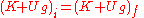Kƒ = 0 because final velocity is zero, and U = 0 because its final distance is infinity, so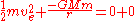Defined a little more formally, "escape velocity" is the initial speed required to go from an initial point in a gravitational potential field to infinity with a residual velocity of zero, with all speeds and velocities measured with respect to the field. Additionally, the escape velocity at a point in space is equal to the speed that an object would have if it started at rest from an infinite distance and was pulled by gravity to that point. In common usage, the initial point is on the surface of a planet
Planet
A planet is a celestial body orbiting a star or stellar remnant that is massive enough to be rounded by its own gravity, is not massive enough to cause thermonuclear fusion, and has cleared its neighbouring region of planetesimals.The term planet is ancient, with ties to history, science,...

or moon
Natural satellite
A natural satellite or moon is a celestial body that orbits a planet or smaller body, which is called its primary. The two terms are used synonymously for non-artificial satellites of planets, of dwarf planets, and of minor planets....

. On the surface of the Earth, the escape velocity is about 11.2 kilometers per second (~6.96 mi/s), which is approximately 34 times the speed of sound (Mach 34) and several times the muzzle velocity
Muzzle velocity
Muzzle velocity is the speed a projectile has at the moment it leaves the muzzle of the gun. Muzzle velocities range from approximately to in black powder muskets , to more than in modern rifles with high-performance cartridges such as the .220 Swift and .204 Ruger, all the way to for tank guns...

of a rifle bullet (up to 1.7 km/s). However, at 9,000 km altitude in "space", it is slightly less than 7.1 km/s.

The escape velocity relative to the surface of a rotating body depends on direction in which the escaping body travels. For example, as the Earth's rotational velocity is 465 m/s at the equator, a rocket launched tangentially from the Earth's equator to the east requires an initial velocity of about 10.735 km/s relative to Earth to escape whereas a rocket launched tangentially from the Earth's equator to the west requires an initial velocity of about 11.665 km/s relative to Earth. The surface velocity decreases with the cosine
Trigonometric function
In mathematics, the trigonometric functions are functions of an angle. They are used to relate the angles of a triangle to the lengths of the sides of a triangle...

of the geographic latitude, so space launch facilities are often located as close to the equator as feasible, e.g. the American Cape Canaveral
Cape Canaveral Air Force Station
Cape Canaveral Air Force Station is an installation of the United States Air Force Space Command's 45th Space Wing, headquartered at nearby Patrick Air Force Base. Located on Cape Canaveral in the state of Florida, CCAFS is the primary launch head of America's Eastern Range with four launch pads...

(latitude 28°28' N) and the French Guiana Space Centre (latitude 5°14' N).

The barycentric escape velocity is independent of the mass of the escaping object. It does not matter if the mass is 1 kg or 1,000 kg, what differs is the amount of energy required. For an object of massthe energy required to escape the Earth's gravitational field is GMm / r, a function of the object's mass (where r is the radius of the Earth, G is the gravitational constant
Gravitational constant
The gravitational constant, denoted G, is an empirical physical constant involved in the calculation of the gravitational attraction between objects with mass. It appears in Newton's law of universal gravitation and in Einstein's theory of general relativity. It is also known as the universal...

, and M is the mass of the Earth
Earth
Earth is the third planet from the Sun, and the densest and fifth-largest of the eight planets in the Solar System. It is also the largest of the Solar System's four terrestrial planets...

, M=5.9736×1024 kg).

For a mass equal to a Saturn V rocket, the escape velocity relative to the launch pad is 8 nanometers per year faster than the escape velocity relative to the mutual center of mass. When the mass reaches the Andromeda galaxy the earth will have recoiled a third of a mile.

## Misconception

Escape velocity is sometimes misunderstood to be the speed a powered vehicle, such as a rocket, must reach to leave orbit and travel through outer space. The quoted escape velocity is commonly the escape velocity at a planet's surface, but it actually decreases with altitude. It is the speed above which an object will depart on a ballistic trajectory, i.e. in free-fall, and never fall back to the surface nor assume a closed orbit. Such an object is said to "escape" the gravity of the planet.

A vehicle with a propulsion system can continue to gain energy and travel away from the planet, in any direction, at a speed lower than escape velocity so long as it is under power. If the vehicle's speed is below the escape velocity and the propulsion is removed, the vehicle will assume a closed orbit or fall back to the surface. If its speed is at or above the escape velocity and the propulsion is removed, it has enough kinetic energy to "escape" and will neither orbit nor fall back to the surface.

## Orbit

If an object attains escape velocity, but is not directed straight away from the planet, then it will follow a curved path. Although this path does not form a closed shape, it is still considered an orbit. Assuming that gravity is the only significant force in the system, this object's speed at any point in the orbit will be equal to the escape velocity at that point (due to the conservation of energy, its total energy must always be 0, which implies that it always has escape velocity; see the derivation above). The shape of the orbit will be a parabola
Parabola
In mathematics, the parabola is a conic section, the intersection of a right circular conical surface and a plane parallel to a generating straight line of that surface...

whose focus is located at the center of mass of the planet. An actual escape requires of course that the orbit not intersect the planet nor its atmosphere, since this would cause the object to crash. When moving away from the source, this path is called an escape orbit. Escape orbits are known as C3
Characteristic energy
In astrodynamics a characteristic energy , a form of specific energy, is a measure of the energy required for an interplanetary mission that requires attaining an excess orbital velocity over an escape velocity required for additional orbital maneuvers...

= 0 orbits (where C3 = −μ/a, and a is the semi-major axis
Semi-major axis
The major axis of an ellipse is its longest diameter, a line that runs through the centre and both foci, its ends being at the widest points of the shape...

).

In reality there are many gravitating bodies in space, so that, for instance, a rocket that travels at escape velocity from Earth will not escape to an infinite distance away because it needs an even higher speed to escape the Sun's gravity. In other words, near the Earth, the rocket's orbit will appear parabolic, but eventually its orbit will become an ellipse around the Sun, except when it is perturbed by the Earth whose orbit it must still intersect.

## List of escape velocitiesLocation with respect to Ve Location with respect to Ve on the SunSunThe Sun is the star at the center of the Solar System. It is almost perfectly spherical and consists of hot plasma interwoven with magnetic fields..., the Sun's gravity: 617.5 km/s on MercuryMercury (planet)Mercury is the innermost and smallest planet in the Solar System, orbiting the Sun once every 87.969 Earth days. The orbit of Mercury has the highest eccentricity of all the Solar System planets, and it has the smallest axial tilt. It completes three rotations about its axis for every two orbits..., Mercury's gravity: 4.3 km/s at Mercury, the Sun's gravity: 67.7 km/s on VenusVenusVenus is the second planet from the Sun, orbiting it every 224.7 Earth days. The planet is named after Venus, the Roman goddess of love and beauty. After the Moon, it is the brightest natural object in the night sky, reaching an apparent magnitude of −4.6, bright enough to cast shadows..., Venus' gravity: 10.3 km/s at Venus, the Sun's gravity: 49.5 km/s on EarthEarthEarth is the third planet from the Sun, and the densest and fifth-largest of the eight planets in the Solar System. It is also the largest of the Solar System's four terrestrial planets..., the Earth's gravity: 11.2 km/s at the Earth/Moon, the Sun's gravity: 42.1 km/s on the MoonMoonThe Moon is Earth's only known natural satellite,There are a number of near-Earth asteroids including 3753 Cruithne that are co-orbital with Earth: their orbits bring them close to Earth for periods of time but then alter in the long term . These are quasi-satellites and not true moons. For more..., the Moon's gravity: 2.4 km/s at the Moon, the Earth's gravity: 1.4 km/s on MarsMarsMars is the fourth planet from the Sun in the Solar System. The planet is named after the Roman god of war, Mars. It is often described as the "Red Planet", as the iron oxide prevalent on its surface gives it a reddish appearance..., Mars' gravity: 5.0 km/s at Mars, the Sun's gravity: 34.1 km/s on JupiterJupiterJupiter is the fifth planet from the Sun and the largest planet within the Solar System. It is a gas giant with mass one-thousandth that of the Sun but is two and a half times the mass of all the other planets in our Solar System combined. Jupiter is classified as a gas giant along with Saturn,..., Jupiter's gravity: 59.5 km/s at Jupiter, the Sun's gravity: 18.5 km/s on SaturnSaturnSaturn is the sixth planet from the Sun and the second largest planet in the Solar System, after Jupiter. Saturn is named after the Roman god Saturn, equated to the Greek Cronus , the Babylonian Ninurta and the Hindu Shani. Saturn's astronomical symbol represents the Roman god's sickle.Saturn,..., Saturn's gravity: 35.6 km/s at Saturn, the Sun's gravity: 13.6 km/s on UranusUranusUranus is the seventh planet from the Sun. It has the third-largest planetary radius and fourth-largest planetary mass in the Solar System. It is named after the ancient Greek deity of the sky Uranus , the father of Cronus and grandfather of Zeus..., Uranus' gravity: 21.2 km/s at Uranus, the Sun's gravity: 9.6 km/s on NeptuneNeptuneNeptune is the eighth and farthest planet from the Sun in the Solar System. Named for the Roman god of the sea, it is the fourth-largest planet by diameter and the third largest by mass. Neptune is 17 times the mass of Earth and is slightly more massive than its near-twin Uranus, which is 15 times..., Neptune's gravity: 23.6 km/s at Neptune, the Sun's gravity: 7.7 km/s on PlutoPlutoPluto, formal designation 134340 Pluto, is the second-most-massive known dwarf planet in the Solar System and the tenth-most-massive body observed directly orbiting the Sun..., Pluto's gravity: 1.229 km/s in the solar systemSolar SystemThe Solar System consists of the Sun and the astronomical objects gravitationally bound in orbit around it, all of which formed from the collapse of a giant molecular cloud approximately 4.6 billion years ago. The vast majority of the system's mass is in the Sun..., the Milky WayMilky WayThe Milky Way is the galaxy that contains the Solar System. This name derives from its appearance as a dim un-resolved "milky" glowing band arching across the night sky...'s gravity: ≥ 525 km/s on the event horizonEvent horizonIn general relativity, an event horizon is a boundary in spacetime beyond which events cannot affect an outside observer. In layman's terms it is defined as "the point of no return" i.e. the point at which the gravitational pull becomes so great as to make escape impossible. The most common case..., a black holeBlack holeA black hole is a region of spacetime from which nothing, not even light, can escape. The theory of general relativity predicts that a sufficiently compact mass will deform spacetime to form a black hole. Around a black hole there is a mathematically defined surface called an event horizon that...'s gravity: = 299,792 km/sSpeed of lightThe speed of light in vacuum, usually denoted by c, is a physical constant important in many areas of physics. Its value is 299,792,458 metres per second, a figure that is exact since the length of the metre is defined from this constant and the international standard for time...

Because of the atmosphere it is not useful and hardly possible to give an object near the surface of the Earth a speed of 11.2 km/s (40,320 km/h), as these speeds are too far in the hypersonic
Hypersonic
In aerodynamics, a hypersonic speed is one that is highly supersonic. Since the 1970s, the term has generally been assumed to refer to speeds of Mach 5 and above...

regime for most practical propulsion systems and would cause most objects to burn up due to atmospheric friction or be torn apart by atmospheric compression. For an actual escape orbit a spacecraft is first placed in low Earth orbit
Low Earth orbit
A low Earth orbit is generally defined as an orbit within the locus extending from the Earth’s surface up to an altitude of 2,000 km...

(160–2,000 km) and then accelerated to the escape velocity at that altitude, which is a little less — about 10.9 km/s. The required change in speed
Delta-v
In astrodynamics a Δv or delta-v is a scalar which takes units of speed. It is a measure of the amount of "effort" that is needed to change from one trajectory to another by making an orbital maneuver....

, however, is far less because from a low Earth orbit the spacecraft already has a speed of approximately 8 km/s.

## Calculating an escape velocity

To expand upon the derivation given in the Overview,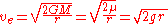whereis the barycentric escape velocity, G is the gravitational constant
Gravitational constant
The gravitational constant, denoted G, is an empirical physical constant involved in the calculation of the gravitational attraction between objects with mass. It appears in Newton's law of universal gravitation and in Einstein's theory of general relativity. It is also known as the universal...

, M is the mass
Mass
Mass can be defined as a quantitive measure of the resistance an object has to change in its velocity.In physics, mass commonly refers to any of the following three properties of matter, which have been shown experimentally to be equivalent:...

of the body being escaped from, r is the distance
Distance
Distance is a numerical description of how far apart objects are. In physics or everyday discussion, distance may refer to a physical length, or an estimation based on other criteria . In mathematics, a distance function or metric is a generalization of the concept of physical distance...

between the center of the body and the point at which escape velocity is being calculated, g is the gravitational acceleration
Gravitational acceleration
In physics, gravitational acceleration is the acceleration on an object caused by gravity. Neglecting friction such as air resistance, all small bodies accelerate in a gravitational field at the same rate relative to the center of mass....

at that distance, and μ is the standard gravitational parameter
Standard gravitational parameter
In astrodynamics, the standard gravitational parameter μ of a celestial body is the product of the gravitational constant G and the mass M of the body.\mu=GM \ The SI units of the standard gravitational parameter are m3s−2....

.

The escape velocity at a given height is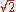times the speed in a circular orbit at the same height, (compare this with equation (14) in circular motion
Circular motion
In physics, circular motion is rotation along a circular path or a circular orbit. It can be uniform, that is, with constant angular rate of rotation , or non-uniform, that is, with a changing rate of rotation. The rotation around a fixed axis of a three-dimensional body involves circular motion of...

). This corresponds to the fact that the potential energy with respect to infinity of an object in such an orbit is minus two times its kinetic energy, while to escape the sum of potential and kinetic energy needs to be at least zero. The velocity corresponding to the circular orbit is sometimes called the first cosmic velocity, whereas in this context the escape velocity is referred to as the second cosmic velocity"

For a body with a spherically-symmetric distribution of mass, the barycentric escape velocityfrom the surface (in m/s) is approximately 2.364×10−5 m1.5kg−0.5s−1 times the radius r (in meters) times the square root of the average density ρ (in kg/m³), or: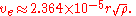## Deriving escape velocity using calculus

These derivations use calculus
Calculus
Calculus is a branch of mathematics focused on limits, functions, derivatives, integrals, and infinite series. This subject constitutes a major part of modern mathematics education. It has two major branches, differential calculus and integral calculus, which are related by the fundamental theorem...

, Newton's laws of motion
Newton's laws of motion
Newton's laws of motion are three physical laws that form the basis for classical mechanics. They describe the relationship between the forces acting on a body and its motion due to those forces...

and Newton's law of universal gravitation
Newton's law of universal gravitation
Newton's law of universal gravitation states that every point mass in the universe attracts every other point mass with a force that is directly proportional to the product of their masses and inversely proportional to the square of the distance between them...

.

### Derivation using only g and r

the Earth's escape speed can be derived from "g
Standard gravity
Standard gravity, or standard acceleration due to free fall, usually denoted by g0 or gn, is the nominal acceleration of an object in a vacuum near the surface of the Earth. It is defined as precisely , or about...

," the barycentric acceleration due to gravity at the Earth's surface. It is not necessary to know the gravitational constant
Gravitational constant
The gravitational constant, denoted G, is an empirical physical constant involved in the calculation of the gravitational attraction between objects with mass. It appears in Newton's law of universal gravitation and in Einstein's theory of general relativity. It is also known as the universal...

G or the mass M of the Earth. Let
r = the Earth's radius, and

g = the barycentric acceleration of gravity at the Earth's surface.

Above the Earth's surface, the acceleration of gravity is governed by Newton's inverse-square
Inverse-square law
In physics, an inverse-square law is any physical law stating that a specified physical quantity or strength is inversely proportional to the square of the distance from the source of that physical quantity....

law of universal gravitation
Newton's law of universal gravitation
Newton's law of universal gravitation states that every point mass in the universe attracts every other point mass with a force that is directly proportional to the product of their masses and inversely proportional to the square of the distance between them...

. Accordingly, the acceleration of gravity at height s above the center of the Earth (where
s > r ) is g (r / s)2.
The weight of an object of mass m at the surface is g m, and its weight at height s above the center of the Earth is gm (r / s)2. Consequently the energy needed to lift an object of mass m from height s above the Earth's center to height s + ds (where ds is an infinitesimal
Infinitesimal
Infinitesimals have been used to express the idea of objects so small that there is no way to see them or to measure them. The word infinitesimal comes from a 17th century Modern Latin coinage infinitesimus, which originally referred to the "infinite-th" item in a series.In common speech, an...

increment of s) is gm (r / s)2 ds. Since this decreases sufficiently fast as s increases, the total energy needed to lift the object to infinite height does not diverge to infinity, but converges to a finite amount. That amount is the integral of the expression above: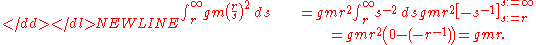That is how much kinetic energy the object of mass m needs in order to escape. The kinetic energy of an object of mass m moving at speed v is (1/2)mv2. Thus we need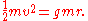The factor m cancels out, and solving for v we get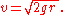If we take the radius of the Earth to be r = 6400 kilometers and the acceleration of gravity at the surface to be g = 9.8 m/s2, we get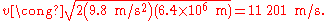This is just a bit over 11 kilometers per second, or a bit under 7 miles per second, as Isaac Newton
Isaac Newton
Sir Isaac Newton PRS was an English physicist, mathematician, astronomer, natural philosopher, alchemist, and theologian, who has been "considered by many to be the greatest and most influential scientist who ever lived."...

calculated.

### Derivation using G and M

Let G be the gravitational constant
Gravitational constant
The gravitational constant, denoted G, is an empirical physical constant involved in the calculation of the gravitational attraction between objects with mass. It appears in Newton's law of universal gravitation and in Einstein's theory of general relativity. It is also known as the universal...

and let M be the mass of the earth and 'm' be the mass of other body to be escaped.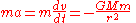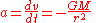By applying the chain rule
Chain rule
In calculus, the chain rule is a formula for computing the derivative of the composition of two or more functions. That is, if f is a function and g is a function, then the chain rule expresses the derivative of the composite function in terms of the derivatives of f and g.In integration, the...

, we get: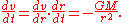Because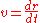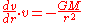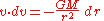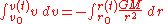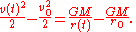Since we want escape velocity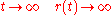and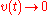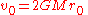v0 is the escape velocity and r0 is the radius of the planet. Note that the above derivation relies on the equivalence of inertial mass and gravitational mass.

### The derivations are consistent

The barycentric gravitational acceleration can be obtained from the gravitational constant G and the mass of Earth M: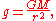where r is the radius of Earth. Thus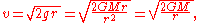so the two derivations given above are consistent.

## Multiple sources

The escape velocity from a position in a field with multiple sources at rest with respect to each other is derived from the total potential energy per kg at that position, relative to infinity. The potential energies for all sources can simply be added. For the escape velocity it can be shown that this gives an escape velocity which is equal to the square root of the sum of the squares of the individual escape velocities due to each source.

For example, at the Earth's surface the escape velocity for the combination Earth and Sun would be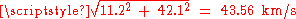.

## Delta-v

To actually achieve escape velocity an object generally needs to apply delta-v
Delta-v
In astrodynamics a Δv or delta-v is a scalar which takes units of speed. It is a measure of the amount of "effort" that is needed to change from one trajectory to another by making an orbital maneuver....

.

However, the required delta-v is reduced by any speed the object may already have, due to its orbital motion, but also notably for objects initially on a planetary surface, there is a significant speed due to the planets rotation.

Where rockets are used to apply the delta-v conservation laws are harder to apply, due to the energy lost and gained by the vehicle from the propellant's initial kinetic energy when forming the exhaust. However, for impulsive maneuvers, the delta-v can be calculated by simply subtracting the vehicle's initial speed from the escape velocity.

## Dynamic situations

The escape velocity calculation assumes that none of the sources of gravitational potential are moving. In the case of the Earth-Moon system, for example, the Moon is in orbit around the Earth, and it turns out that an object that passes behind the moon can gain speed from the encounter (a gravitational assist).

Therefore, there are certain launch angles and launch window
Launch window
Launch window is a term used in spaceflight to describe a time period in which a particular launch vehicle must be launched. If the rocket does not launch within the "window", it has to wait for the next window....

s that will give the assist. This will give an object extra speed in-flight and still permit it to escape Earth's gravity, even though it was launched with less than escape velocity.

## Gravity well

In the hypothetical case of uniform density, the velocity that an object would achieve when dropped in a hypothetical vacuum hole from the surface of the Earth to the center of the Earth is the escape velocity divided by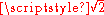, i.e. the speed in a circular orbit at a low height. Correspondingly, the escape velocity from the center of the Earth would be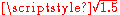times that from the surface.

A refined calculation would take into account the fact that the Earth's mass is not uniformly distributed near its core. This gives higher speeds.

## See also

• Orbital speed
Orbital speed
The orbital speed of a body, generally a planet, a natural satellite, an artificial satellite, or a multiple star, is the speed at which it orbits around the barycenter of a system, usually around a more massive body...

• Characteristic energy
Characteristic energy
In astrodynamics a characteristic energy , a form of specific energy, is a measure of the energy required for an interplanetary mission that requires attaining an excess orbital velocity over an escape velocity required for additional orbital maneuvers...

(C3)
• Delta-v budget
Delta-v budget
In the astrodynamics and aerospace industry, a delta-v budget is the estimated delta-v requirements for the various propulsive tasks and orbital maneuvers over one or more phases of a space mission.Sample delta-v budget will enumerate various classes of maneuvers, delta-v per maneuver, number of...

– speed needed to perform manoeuvres.
• Gravitational slingshot – a technique for changing trajectory.
• Gravity well
Gravity well
A gravity well or gravitational well is a conceptual model of the gravitational field surrounding a body in space. The more massive the body the deeper and more extensive the gravity well associated with it. The Sun has a far-reaching and deep gravity well. Asteroids and small moons have much...

• Two-body problem
Two-body problem
In classical mechanics, the two-body problem is to determine the motion of two point particles that interact only with each other. Common examples include a satellite orbiting a planet, a planet orbiting a star, two stars orbiting each other , and a classical electron orbiting an atomic nucleus In...

• Black hole
Black hole
A black hole is a region of spacetime from which nothing, not even light, can escape. The theory of general relativity predicts that a sufficiently compact mass will deform spacetime to form a black hole. Around a black hole there is a mathematically defined surface called an event horizon that...

– an object with an escape velocity greater than the speed of light
• Oberth effect
Oberth effect
In astronautics, the Oberth effect is where the use of a rocket engine when travelling at high speed generates much more useful energy than one at low speed...

– burning fuel deep in a gravity field gives higher change in velocity.
• Newton's cannonball
Newton's cannonball
Newton's cannonball was a thought experiment Isaac Newton used to hypothesize that the force of gravity was universal, and it was the key force for planetary motion...

• List of artificial objects escaping from the Solar System
• List of artificial objects in heliocentric orbit

## External links

The source of this article is wikipedia, the free encyclopedia.  The text of this article is licensed under the GFDL.